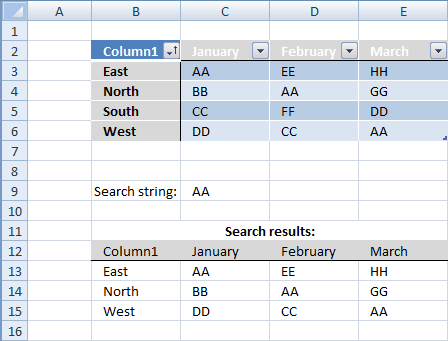Author: Oscar Cronquist Article last updated on November 21, 2018
Question:
How do I identify rows that have a specific cell value in an Excel defined table?### Array formula in B13:E15

=IFERROR(INDEX(Table1, SMALL(IF(Table1=\$C\$9, ROW(Table1)-MIN(ROW(Table1))+1, ""), ROW(Table1)-MIN(ROW(Table1))+1), COLUMN(Table1)-MIN(COLUMN(Table1))+1), "")

#### How to create an array formula

1. Select cell range B13:E15
2. Copy/Paste above formula
3. Press and hold Ctrl + Shift
4. Press enter once
5. Release all keys

#### Excel defined table

Table1 (B3:E6)
What is named ranges?

### Array formula explanation

Step 1 - Find cells in named range matching criterion

=IFERROR(INDEX(Table1, SMALL(IF(Table1=\$C\$9, ROW(Table1)-MIN(ROW(Table1))+1, ""), ROW(Table1)-MIN(ROW(Table1))+1), COLUMN(Table1)-MIN(COLUMN(Table1))+1), "")

Table1=\$C\$9

becomes

B3:E6=\$C\$9

becomes

{East, AA, EE, HH; North, BB, AA, GG; South, CC, FF, DD; West, DD, CC, AA}= AA

becomes

{FALSE,TRUE,FALSE,FALSE,FALSE,FALSE,TRUE,FALSE,FALSE, FALSE,FALSE,FALSE,FALSE,FALSE,FALSE,TRUE}

Step 2 - Extract row numbers

=IFERROR(INDEX(Table1, SMALL(IF(Table1=\$C\$9, ROW(Table1)-MIN(ROW(Table1))+1, ""), ROW(Table1)-MIN(ROW(Table1))+1), COLUMN(Table1)-MIN(COLUMN(Table1))+1), "")

IF(Table1=\$C\$9, ROW(Table1)-MIN(ROW(Table1))+1, "")

becomes

IF({FALSE,TRUE,FALSE,FALSE,FALSE,FALSE,TRUE,FALSE,FALSE, FALSE,FALSE,FALSE,FALSE,FALSE,FALSE,TRUE}, {1,1,1,1;2,2,2,2;3,3,3,3;4,4,4,4}, "")

becomes

{"",1,"","";"","",2,"";"","","","";"","","",4}

Step 3 - Return the k-th smallest row number

SMALL(array,k) returns the k-th smallest number in this data set.

SMALL(IF(Table1=\$C\$9, ROW(Table1)-MIN(ROW(Table1))+1, ""), ROW(Table1)-MIN(ROW(Table1))+1)

becomes

SMALL({"",1,"","";"","",2,"";"","","","";"","","",4}, {1,1,1,1;2,2,2,2;3,3,3,3;4,4,4,4})

becmoes

{1,1,1,1;2,2,2,2;4,4,4,4}

Step 4 - Return matching records

INDEX(Table1, SMALL(IF(Table1=\$C\$9, ROW(Table1)-MIN(ROW(Table1))+1, ""), ROW(Table1)-MIN(ROW(Table1))+1), COLUMN(Table1)-MIN(COLUMN(Table1))+1)

becomes

INDEX(Table1, {1,1,1,1;2,2,2,2;4,4,4,4}, COLUMN(Table1)-MIN(COLUMN(Table1))+1)

becomes

INDEX(Table1, {1,1,1,1;2,2,2,2;4,4,4,4}, {1,2,3,4;1,2,3,4;1,2,3,4;1,2,3,4})

and returns:

{"East","AA","EE","HH";"North","BB","AA","GG";"West","DD","CC","AA"}

Get Excel *.xlsx file
search-for-a-string-in-excel-table.xlsx